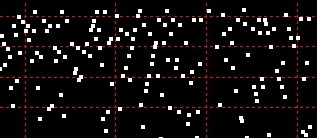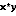Compositeshome

 Lists of Composites and Composite Generators with Prime FactorsView in your browser, download files, or generate your own 4 = 2 * 2 6 = 2 * 3 8 = 2 * 2 * 2 9 = 3 * 3 10 = 2 * 5 12 = 2 * 2 * 3 14 = 2 * 7 15 = 3 * 5 16 = 2 * 2 * 2 * 2 18 = 2 * 3 * 3 20 = 2 * 2 * 5 21 = 3 * 7 22 = 2 * 11 24 = 2 * 2 * 2 * 3 25 = 5 * 5 26 = 2 * 13 27 = 3 * 3 * 3 28 = 2 * 2 * 7 30 = 2 * 3 * 5 32 = 2 * 2 * 2 * 2 * 2 33 = 3 * 11 34 = 2 * 17 35 = 5 * 7 36 = 2 * 2 * 3 * 3 38 = 2 * 19 39 = 3 * 13 40 = 2 * 2 * 2 * 5 42 = 2 * 3 * 7 44 = 2 * 2 * 11 45 = 3 * 3 * 5 46 = 2 * 23 48 = 2 * 2 * 2 * 2 * 3 49 = 7 * 7 50 = 2 * 5 * 5 51 = 3 * 17 52 = 2 * 2 * 13 54 = 2 * 3 * 3 * 3 55 = 5 * 11 56 = 2 * 2 * 2 * 7 57 = 3 * 19 58 = 2 * 29 60 = 2 * 2 * 3 * 5 62 = 2 * 31 63 = 3 * 3 * 7 64 = 2 * 2 * 2 * 2 * 2 * 2 65 = 5 * 13 66 = 2 * 3 * 11 68 = 2 * 2 * 17 69 = 3 * 23 70 = 2 * 5 * 7 72 = 2 * 2 * 2 * 3 * 3 74 = 2 * 37 75 = 3 * 5 * 5 76 = 2 * 2 * 19 77 = 7 * 11 78 = 2 * 3 * 13 80 = 2 * 2 * 2 * 2 * 5 81 = 3 * 3 * 3 * 3 82 = 2 * 41 84 = 2 * 2 * 3 * 7 85 = 5 * 17 86 = 2 * 43 87 = 3 * 29 88 = 2 * 2 * 2 * 11 90 = 2 * 3 * 3 * 5 91 = 7 * 13 92 = 2 * 2 * 23 93 = 3 * 31 94 = 2 * 47 95 = 5 * 19 96 = 2 * 2 * 2 * 2 * 2 * 3 98 = 2 * 7 * 7 99 = 3 * 3 * 11 100 = 2 * 2 * 5 * 5 These are comma-delimited text files containing the composite number and its prime factors. First 100 1 - 133  (2KB) First 1,000 1 - 1,197  (20KB) First 10,000 1 - 11,374  (217KB) First 100,000 1 - 110,487 (2.5MB) First 1,000,000 1 - 1,084,605 (6.5MB - zip download only)   Want More Composites and Their Prime Factors...? Generate lists of composites and their prime factors up to 10^15 (1 quadrillion).Download Prime Factor Generator (13KB)   Want Distinct Prime Factors Only...? Generate distinct prime factors and the number of distinct factors.For example, the complete prime factorization of 1000000 is: 2 * 2 * 2 * 2 * 2 * 2 * 5 * 5 * 5 * 5 * 5 * 5 The distinct prime factors are 2 and 5, and the number of distinct factors is 2. The number of distinct prime factors is the subject of the Erdős–Kac theorem. They are denoted ω(n). So ω(1000000) = 2. New and Improved Version Additions by Klaus Q. Fischer This new version has several improvements. The most notable change is that it shows the exponent for each prime factor. So, for example, the distinct factors of 500 are now represented thus: 500: 2 = 2,2 * 5,3 This can be read as "500 has 2 distinct factors: 2 (squared) and 5 (cubed)". (The full notation would be 2 * 2 * 5 * 5 * 5.) Output composites and their prime factors in a selected range to text files. Save the program (PFactors.exe or DFactors.exe) to a folder. Specify From and To values. Select the range (number of values*) for each text file. Click Start. What you get? PFactors.exe The first number is the composite and the remaining numbers are its prime factors. (For example, 693 = 11 * 7 * 3 * 3 shows the unique prime factorization of 693. The same factor is shown as many time as required to create the sum.) DFactors2.exe (new version) The first number is the composite; the second number is the number of distinct prime factors; the pairs of comma-separated numbers are the distinct prime factors and their exponents. (For example, 693: 3 = 3,2 * 7,1 * 11,1 means that for 693 there are 3 distinct prime factors and that prime factor 3 is squared.) DFactors.exe (original version) The first number is the composite; the comma-separated numbers are the distinct prime factors; the third number is the number of distinct factors. (For example, 693: 3,7,11 : 3 means that for 693 there are 3 distinct prime factors.) *If you accept the default of 100,000, that means 100,000 values (and lines) will be written to each file.

© 2012 Michael M. Ross

Secrets of the Spiral